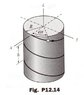# Pytels Dynamics 12.14: particle moves on helix

Alexanddros81

## Homework Statement

When a particle moves along the helix shown, the componentsof its position vector are
x=Rcosωt , y = Rsinωt , ##z=-\frac h {2π} ωt##

where ω is constant. Show that the velocity and acceleration have constant magnitutes,
and compute their values if R=1.2m, h=0.75m, and ω=4π rad/s

## The Attempt at a Solution

for a start I would like someone to tell me by computing vz = dz/dhxdh/dt
what dh/dt is equal to? Since h = 0.75m = constant, then vz=0 and az = 0
Is my asumption correct?

#### Attachments

•12_14 Pytel.jpg
41.3 KB · Views: 439